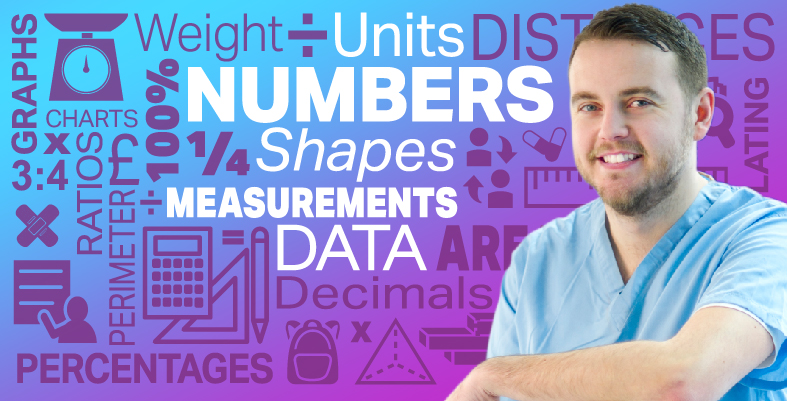Everyday maths for Health and Social Care and Education Support 1

Start this free course now. Just create an account and sign in. Enrol and complete the course for a free statement of participation or digital badge if available.

Free course

# 3.1 Weighing things

It’s useful to have an idea of how much things weigh. It can help you to work out the weight of fruit or vegetables so that you eat the correct 5-a-day portion size or to find out whether a patient is a healthy weight.

Try estimating the weight of something before you weigh it. It will help you to get used to measures of weight.

Hint: Remember to use appropriate units. Give the weight of small things in grams and of heavy things in kilograms.

Take a look at the example below before having a go at the activity.

## Example: Weighing a person

1. Which metric unit would you use to measure the weight of a person?
2. Estimate how much you, or someone at home, weighs. Then use a set of scales for an exact measurement.
3. How much would 20 people of a similar size weigh altogether? Would you use the same units?

### Method

1. The most common units used to measure a person’s weight are stones and pounds or kilograms. Kilograms are the metric unit used.
2. How much did you estimate that a person weighs? A healthy weight for an adult is usually somewhere in the range of 38 to 108 kg. A healthy weight of a person depends on many different factors such as height and sex.

When we weighed a person, they weighed 80 kg.

3. Twenty people of a similar size would weigh:

• 80 × 20 = 1,600 kg in total

Remember the metric conversion diagram? To convert from kilograms to metric tonnes, you need to divide the figure in kilograms by 1,000. So the weight of the people in metric tonnes is:

• 1,600 kg ÷ 1,000 = 1.6 tonnes

Now try the following activity. Remember to check your answers once you have completed the questions.

## Activity 7: Weighing things

1. How much do ten teabags weigh? Estimate and then weigh them.
2. How heavy is a bottle of sauce? How much would a case of 10 bottles weigh?

Hint: The weight shown on the label is the weight of the sauce – it doesn’t include the weight of the bottle or jar that the sauce comes in. So for an accurate measurement, you need to weigh the bottle rather than read the label!

3. How heavy is a book?

### Discussion

Our suggestions are shown in the table below. Your estimates and measured weights might be different, but they should be roughly similar.

ItemEstimated weightActual weight
Ten teabags25 g30 g
Bottle of sauce500 g450 g
Book900 g720 g

A case of ten bottles of sauce would weigh:

• 450 × 10 = 4,500 g

As previously noted, 1,000 g = 1 kg, so 4,500 g = 4.5 kg – which is how you would more usually express this weight.

If your book weighed more than ours, you might have given its weight in kilograms. If you chose a small book, it may have weighed a lot less.

FSM_SSH_1